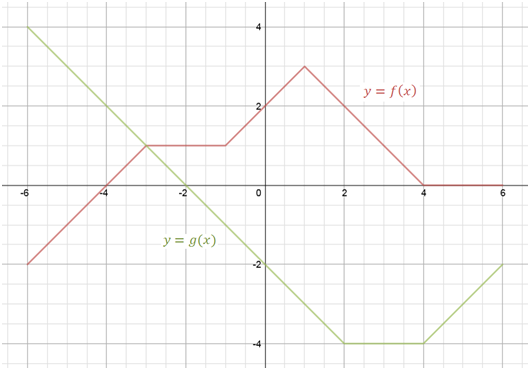# Adding functions

### Adding functions

#### Lessons

adding functions: $(f+g)(x) = f(x)+g(x)$
• 1.
Determine the Sum of Two Functions Algebraically
If $f(x)= 5x^3-x+6$ and $g(x)=4x-8$, determine:
a)
$(f+g)(x)$

b)
$(f+g)(2)$, evaluate in two different ways

• 2.
Determine the Sum of Two Functions Graphically
Given the graphs of functions $f(x)$ and $g(x)$a)
sketch: $(f+g)(x)$

b)
evaluate:
i)
$(f+g)(-5)$
ii)
$(f+g)(0)$
iii) $(f+g)(5)$

• 3.
Determine the Value of a Combined Function
If $f(x)=\sqrt{2x}-1$ and $g(x) = -|6-x|$
Evaluate: $5(f+g)(8)-10$